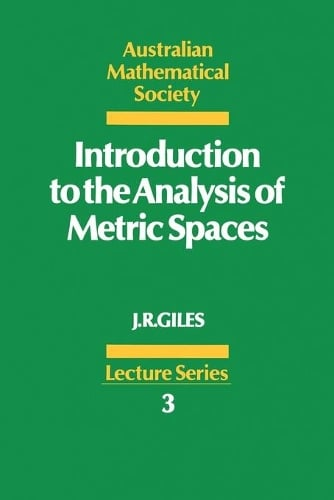•# Introduction to the Analysis of Metric Spaces - Australian Mathematical Society Lecture Series (Paperback)

(author)
£40.99
Paperback 272 Pages / Published: 03/09/1987
• We can order this

Usually dispatched within 3 weeks

This is an introduction to the analysis of metric and normed linear spaces for undergraduate students in mathematics. Assuming a basic knowledge of real analysis and linear algebra, the student is exposed to the axiomatic method in analysis and is shown its power in exploiting the structure of fundamental analysis, which underlies a variety of applications. An example is the link between normed linear spaces and linear algebra; finite dimensional spaces are discussed early. The treatment progresses from the concrete to the abstract: thus metric spaces are studied in some detail before general topology is begun, though topological properties of metric spaces are explored in the book. Graded exercises are provided at the end of each section; in each set the earlier exercises are designed to assist in the detection of the structural properties in concrete examples while the later ones are more conceptually sophisticated.

Publisher: Cambridge University Press
ISBN: 9780521359283
Number of pages: 272
Weight: 410 g
Dimensions: 231 x 153 x 17 mm